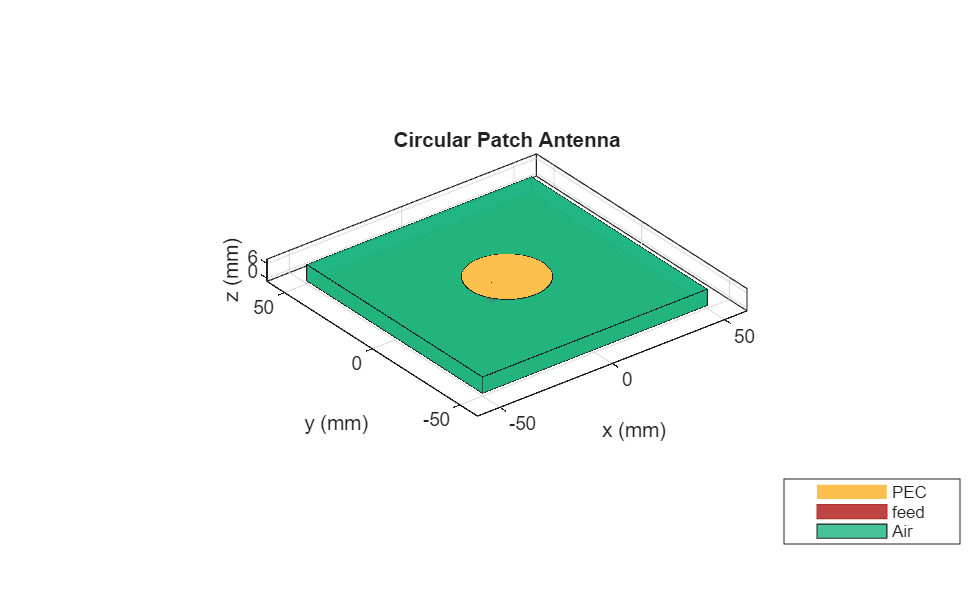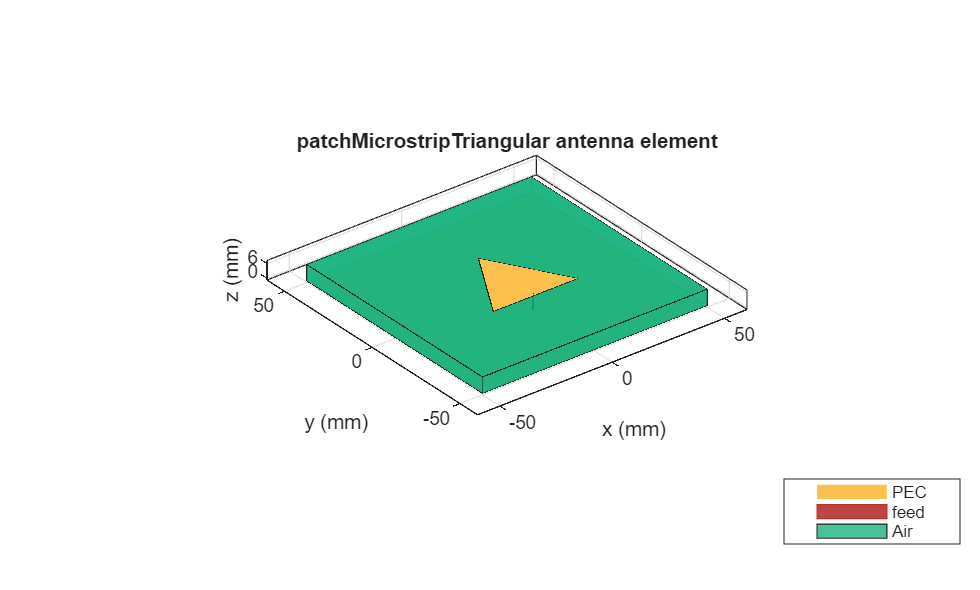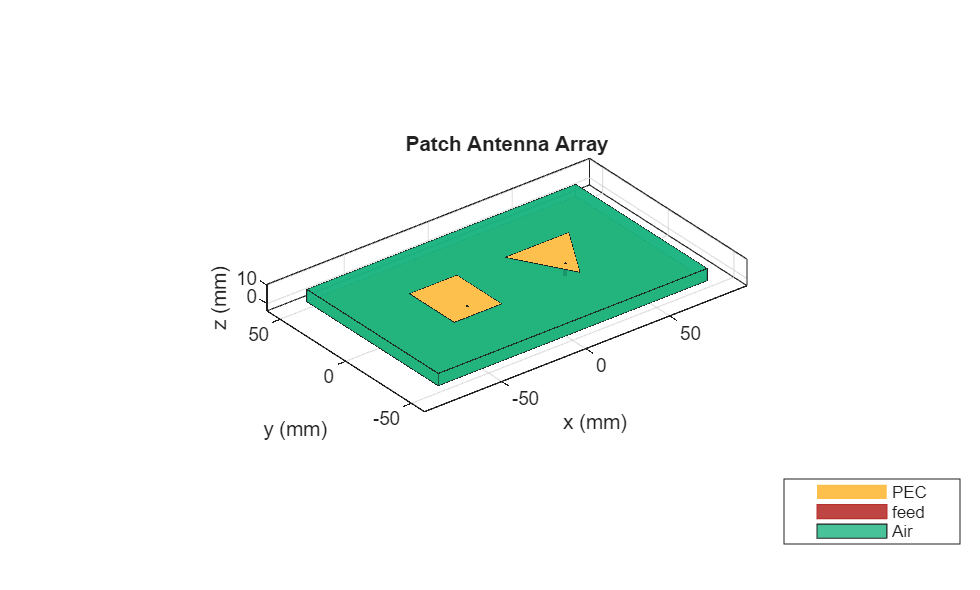# ISM Band Patch Microstrip Antennas and Mutual Coupling Between different patches

This example shows how to design and implement a rectangular, circular, triangular and elliptical patch microstrip antennas complying with the ISM (Industrial Scientific and Medical) band.

### Define Parameters

All these microstrip antennas consisting of 6.6mm moderately thick and FR4 substrate of 4.2 EpsilonR, 0.02 loss tangent and square ground plane of 100mm x 100mm, fed by 1.3mm diameter coaxial probes, are designed to comply with the ISM band (2.4 - 2.5GHz).

```LGp = 100e-3; % Ground plane Length WGp = 100e-3; % Ground plane width h = 6.6e-3; % Height of the substrate ```

### Define Parameters Of Elliptical Patch Antenna

Design a probe feed patch micro strip elliptical antenna using a dimension of 33.5mm major axis, 18.8mm minor axis. The feed is offset by 11.6mm from the origin along the x axis.

```a = 33.5e-3; % Major Axis b = 18.8e-3; % Minor Axis f = 11.6e-3; % Feed Offset d = dielectric('EpsilonR',4.2,'LossTangent',0.02); ```

Create a patch micro strip Elliptical antenna using the defined parameters

```p_Ellipse= patchMicrostripElliptical('MajorAxis',a,'MinorAxis',b,... 'Height',h,'Substrate',d,'GroundPlaneLength',LGp,'GroundPlaneWidth',... WGp,'FeedOffset',[-(a/2-f) 0]); figure; show(p_Ellipse); ```### Define Parameters Of Circular Patch Antenna

Design a probe feed circular patch micro strip antenna using a dimension of 16mm Radius. The feed is offset by 9.25mm from the origin along the x axis.

```r = 16e-3; % Radius f1 = 9.25e-3; % Feed Offset ```

Create a patch micro strip Circular antenna using the defined parameters

```p_Circle= patchMicrostripCircular('Radius',r,'Height',h,'Substrate',d,... 'GroundPlaneLength',LGp,'GroundPlaneWidth',WGp,'FeedOffset',[-(r-f1) 0]); figure; show(p_Circle); ```### Define Parameters Of Rectangular Patch Antenna

Design a probe feed Rectangular patch micro strip antenna using a dimension of 28.20mm Length, 34.06mm width. The feed is offset by 5.3mm from the origin along the x axis.

```rect1 = 28.20e-3; % Length rect2 = 34.06e-3; % width f2 = 5.3e-3; % feedoffset ```

Create a patch micro strip Rectangular antenna using the defined parameters

```p_rect= patchMicrostrip('Length',rect1,'Width',rect2,'Height',h,'Substrate',d,... 'GroundPlaneLength',LGp,'GroundPlaneWidth',WGp,... 'FeedOffset',[-(rect1/2-f2) 0]); figure; show(p_rect); ```### Define Parameters Of Triangular Patch Antenna

Design an equilateral Triangular patch micro strip antenna using a dimension of 37.63mm side. The feed is offset by 3.8mm from the origin along the y axis.

```side = 37.63e-3; f_off = 3.8e-3; p_triang= patchMicrostripTriangular('side',side,'Height',h,'Substrate',d,... 'GroundPlaneLength',LGp,'GroundPlaneWidth',WGp,... 'FeedOffset',[0 -side/2+f_off]); figure; show(p_triang); ```### Visualize The Reflection Coefficient Magnitude Of The Patches

Plot the reflection coefficient for these antennas over the band and a reference impedance of 50 ohms. Curves for the reflection coefficient magnitude are shown in the below figure. we did the manual mesh of patch antennas with different edge lengths.The directivity of the antennas are around 6.37dB for Elliptical patch, 7dB for Circular patch, 7.37dB for Rectangular patch and 6.16dB for Triangular patch.### Mutual Coupling Between Rectangular and Triangular Patches

This section is devoted to the study of cases, focusing on configurations with the lowest mutual couplings. The relative displacement 'd' is fixed as lambda/2, two patches placed side-by-side configuration and the patches positioned in the center of the rectangular 160mm x 100mm ground plane with a substrate of FR4.

```LGp1 = 160e-3; % Ground plane Length WGp1 = 100e-3; % Ground plane width Ground_plane1=antenna.Rectangle('Length',LGp1,'Width',WGp1);% Ground plane dis = 0.0612; % distance between two patches (d = lambda/2) ```

Create a patch micro strip Rectangular antenna using the defined parameters

```r_ant = pcbStack(p_rect); rect_p = r_ant.Layers{1}; rect_p.Center = [-dis/2 0]; ```

Create a patch micro strip Triangular antenna using the defined parameters

```t_ant = pcbStack(p_triang); triangle_p = t_ant.Layers{1}; triangle_p= rotateZ(triangle_p,180); triangle_p= translate(triangle_p,[dis/2, 0, 0]); patch = rect_p+triangle_p; % adding patches ```

### Define PCB Stack

Use the pcb Stack to define the metal and dielectric layers for mutual coupling patch antenna. the top-most layer is a patch layer and the second layer is dielectric layer and the third layer is the ground plane.

```p_mc=pcbStack; d4=dielectric('EpsilonR',4.2,'Thickness',h,'LossTangent',0.02); p_mc.BoardThickness=d4.Thickness; p_mc.BoardShape.Length=LGp1; p_mc.BoardShape.Width=WGp1; p_mc.Layers={patch,d4,Ground_plane1}; p_mc.FeedLocations=[-dis/2 -(rect1/2-f2) 1 3; dis/2 -5.3075e-3 1 3]; p_mc.FeedDiameter=1.3e-3; figure; show(p_mc); ```### Radiation Pattern Of Mutual Coupling Patches

The side-by -side configuration directivity is 7.4dB

```figure; pattern(p_mc,2.45e9); ```### Pattern Magnitude

```figure;[fm,~,t10] = pattern(p_mc,2.45e9,0,0:360); polarpattern(t10,fm); ```### Reference

2) Probe - Fed Linearly - Polarized Electrically Equivalent Microstrip Antennas on FR4 Substrates